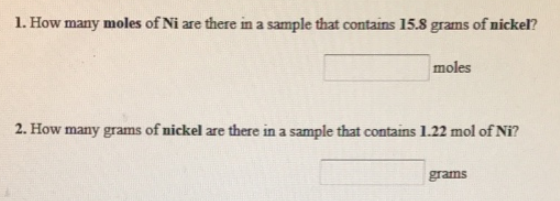# Problem: 1. How many moles of Ni are there in a sample that contains 15.8 grams of nickel? 2. How many grams of nickel are there in a sample that contains 1.22 mol of Ni?

###### FREE Expert Solution

Use the mole concept and atomic mass of Ni to calculate mass and moles vice versa

88% (280 ratings)###### Problem Details

1. How many moles of Ni are there in a sample that contains 15.8 grams of nickel?

2. How many grams of nickel are there in a sample that contains 1.22 mol of Ni?